## Plane

A plane is a 2-D Surface spanned by two linearly independent vectors. The generalization of the plane to higher Dimensions is called a Hyperplane.

In intercept form, a plane passing through the points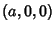,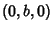and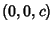is given by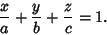(1)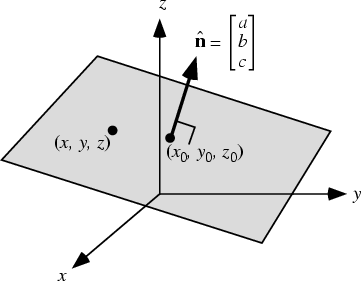The equation of a plane Perpendicular to the Nonzero Vector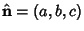through the point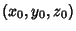is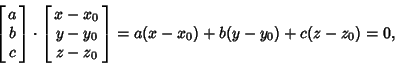(2)

so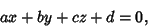(3)

where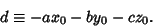(4)

A plane specified in this form therefore has-,-, and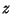-intercepts at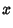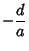(5)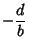(6)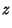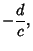(7)

and lies at a Distance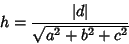(8)

from the Origin.

The plane through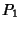and parallel to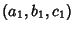and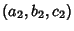is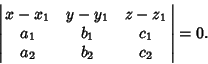(9)

The plane through pointsand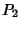parallel to direction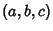is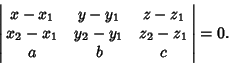(10)

The three-point form is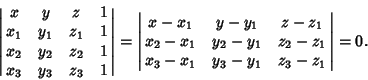(11)

The Distance from a point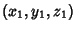to a plane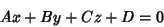(12)

is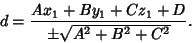(13)

The Dihedral Angle between the planes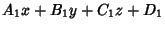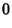(14)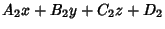(15)

is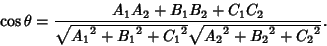(16)

In order to specify the relative distances of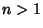points in the plane,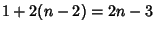coordinates are needed, since the first can always be placed at (0, 0) and the second at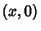, where it defines the x-Axis. The remaining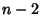points need two coordinates each. However, the total number of distances is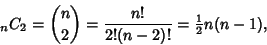(17)

where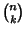is a Binomial Coefficient, so the distances between points are subject torelationships, where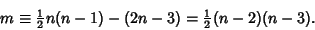(18)

For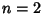and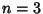, there are no relationships. However, for a Quadrilateral (with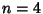), there is one (Weinberg 1972).

It is impossible to pick random variables which are uniformly distributed in the plane (Eisenberg and Sullivan 1996). In 4-D, it is possible for four planes to intersect in exactly one point. For every set ofpoints in the plane, there exists a point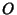in the plane having the property such that every straight line throughhas at least 1/3 of the points on each side of it (Honsberger 1985).

Every Rigid motion of the plane is one of the following types (Singer 1995):

1. Rotation about a fixed point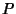.

2. Translation in the direction of a line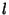.

3. Reflection across a line.

4. Glide-reflections along a line.

Every Rigid motion of the hyperbolic plane is one of the previous types or a

5. Horocycle rotation.

See also Argand Plane, Complex Plane, Dihedral Angle, Elliptic Plane, Fano Plane, Hyperplane, Moufang Plane, Nirenberg's Conjecture, Normal Section, Point-Plane Distance, Projective Plane

References

Beyer, W. H. CRC Standard Mathematical Tables, 28th ed. Boca Raton, FL: CRC Press, pp. 208-209, 1987.

Eisenberg, B. and Sullivan, R. Random TrianglesDimensions.'' Amer. Math. Monthly 103, 308-318, 1996.

Honsberger, R. Mathematical Gems III. Washington, DC: Math. Assoc. Amer., pp. 189-191, 1985.

Singer, D. A. Isometries of the Plane.'' Amer. Math. Monthly 102, 628-631, 1995.

Weinberg, S. Gravitation and Cosmology: Principles and Applications of the General Theory of Relativity. New York: Wiley, p. 7, 1972.